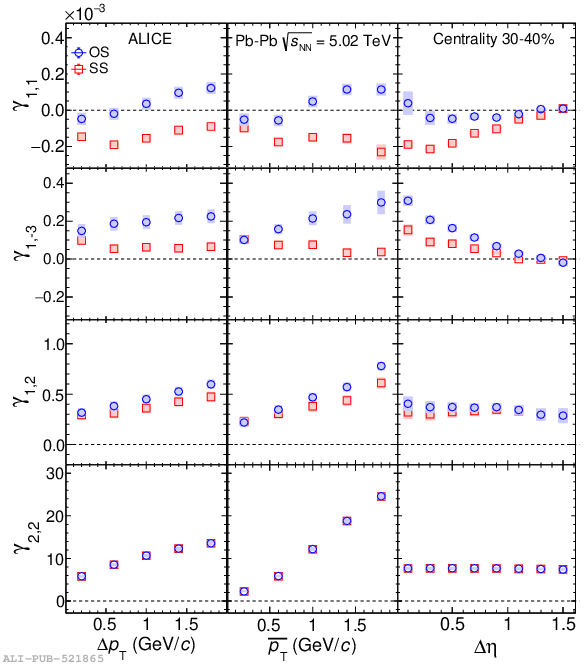# Figure 4

 The dependence of $\gamma_{1,1}$, $\gamma_{1,-3}$, $\gamma_{1,2}$ and $\gamma_{2,2}$ on the transverse momentum difference $\Delta p_{\mathrm{T}} = |p_{\mathrm{T},\alpha} - p_{\mathrm{T},\beta}|$ (left panel), the average transverse momentum $\overline{p}_{\mathrm{T}} = (p_{\mathrm{T},\alpha} + p_{\mathrm{T},\beta})/2$ (middle panel) and the pseudorapidity difference $\Delta\eta = |\eta_{\alpha} - \eta_{\beta}|$ (right panel) of the pair. The results for both opposite and same sign particle pairs are reported for one indicative centrality interval (30--40$\%$) of Pb--Pb collisions at $\sqrt{s_\mathrm{{\rm NN}}} = 5.02$ TeV.